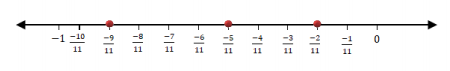# Important Questions Class 8 Maths Chapter 1 Rational Numbers

Important questions for class 8 chapter 1- rational numbers are given here. These questions include short answer type questions and long answer type questions related to rational numbers which are important for class 8 maths examination. To score good marks in the exam, students can practice these questions which are based on NCERT syllabus.

Rational numbers are the numbers which can be represented in the form of p/q, where p and q are integers, and q is not equal to 0. Let us check here what are the important questions related to this chapter.

Also Check:

## Rational Numbers Important Questions For Class 8

The questions from rational numbers are segregated into short and long answer type questions.

1. What are the multiplicative and additive identities of rational numbers?

Solution: 0 and 1 are the additive and multiplicative identity of rational numbers respectively.

2. Write the additive inverse of 19/-6 and -⅔

Solution: 19/-6 = 19/6 and -⅔ = 2/3

3. Write the multiplicative inverse of -13/19 and -7

Solution: -13/19 = -19/13 and -7 = -1/7

4. Mention a rational number which has no reciprocal.

Solution: A rational number “0” has no reciprocal or multiplicative inverse.

5. Mention any 4 rational numbers which are less than 5.

Solution: -1, 1, 2 and 3.

6. Mention the commutativity, associative and distributive properties of rational numbers. Also, check a × b = b × a and a + b = b + a for a = ½ and b = ¾

Solution:

Commutative property:
For any two rational numbers a and b, a + b = b + a.
For any two rational numbers a and b, a × b = b × a.
Associative Property:
For any three rational numbers a, b and c,
(a + b) + c = a + (b + c)
Distributive property states that for any three numbers x, y and z,
x × ( y + z ) = (x × y) + ( x × z)

a*b = b*a
a*b = ½ * ¾ = 3/8
b*a = ¾ * ½ = 3/8
a + b = ¾ + ½ = 5/4
b + a = ½ + ¾ = 5/4

7. Write any 5 rational numbers between −2/5 and ½.

Solution:
−2/5 can be written as −8/20.
1/2 can be written as 10/20.
So, rational numbers between these two numbers can be,
−7/20,−6/20,−5/20,−4/20,−3/20,−2/20,−1/20,0,1/20,2/20,3/20,4/20.

8. If the product of any two rational numbers is 2 and one of them is 1/7, find the other?

Solution: Consider 2 rational numbers as “a” and “b”.

Given, a = 1/7 and a × b = 2

Now, 1/7 × b = 2

⇒ b = 7 × 2 = 14

So, the other rational number will be 14.

9. Mr X went shopping with a certain amount of money. He spent Rs. 10(¼) on buying a pen and Rs. 25(¾) in food. He then gave the remaining Rs. 16(½) to his friend. Calculate how much money he initially had.

Solution: To get the amount of money Mr X had initially, his purchases have to be added.

So,

Initial Money = 10(¼) + 25(¾) + 16(½)

= 41/4 + 103/4 + 33/2

By taking LCM, we get

Initial Money = 105/2

10. Represent −𝟐/𝟏𝟏, −𝟓/𝟏𝟏, and −𝟗/ 𝟏𝟏 on the number line.

Solution: To represent these numbers, divide the number line into 11 parts. Now, the given rational numbers will be 2, 5 and 9 points away from 0.### Extra Practice Questions For Class 8 Maths Chapter 1 Rational Numbers

1. Give three rational numbers between 3/6 and 3/4.

2. What is the property used for the expression given  -2/3 × 3/5 + 5/2 -3/5 × 1/6 ?

3.  Give 2 rational numbers whose multiplicative inverse is same as they are.

4. Give a rational number that is equivalent to 4/7 with
(a) numerator 20
(b) denominator 28

5. What is the additive inverse of 3/4?
(a) -3/4
(b) 4/3
(c) -4/3
(d) -2/3

### Articles Related to Class 8 Rational Numbers

Keep visiting BYJU’S to get more such maths resources and materials. Also, download BYJU’S- The Learning App to learn various math concepts in a more personalized, engaging and efficient way.

1. anamika singh

where can i get solving questions just for the properties

2. 10 no. Question is a question

3. Potharju Deeraj

Hi sir

4. This app is amazing

5. This is awesome app

6. prapti mehta

this app is amazing and helps us in learning new things and new methods.

7. Vedant Sharma

This Helped me alot

8. Prabhgun singh

TThis really helped me

1. Aashika Reddy

yes this helped for exams

9. Thanks for your wonderful effort! This actually helped me a lot!